# Chapter 1 : Numbers and Operations

### Topics covered in this snack-sized chapter:

#### Numbers arrow_upward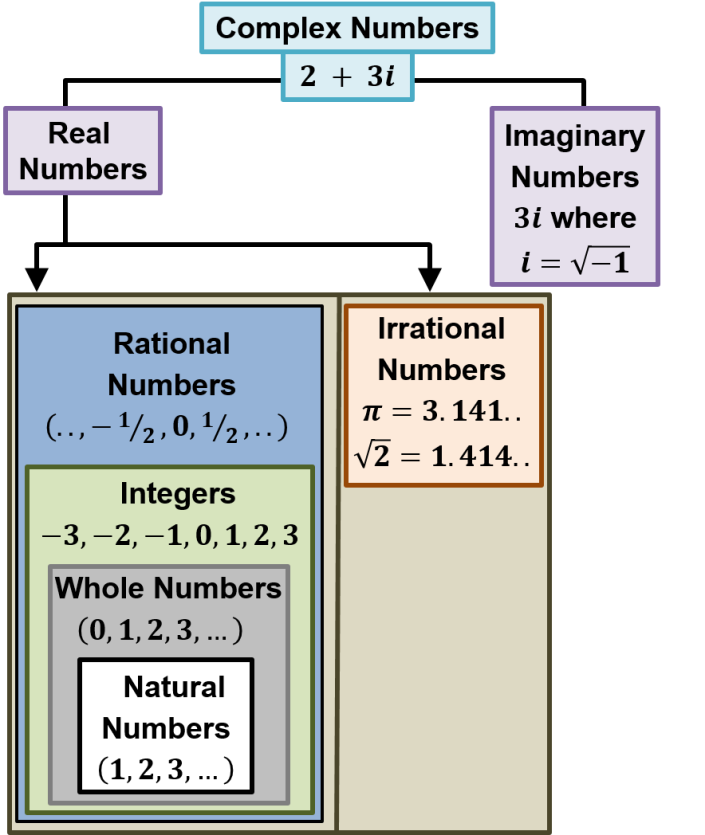Natural Numbers

• The natural numbers are the counting numbers.
• Examples: 1, 2, 3, 4, 5.
• Whole Numbers

• The whole numbers are the counting numbers including 0.
• Examples:  0, 1, 2, 3, 4, 5.
• Integers

• Integers include counting numbers, zero and negative numbers.
• Examples: -3, -2, -1, 0, 1, 2, 3.
• Rational Number

• A rational number is a number that can be expressed as a fraction or ratio.
• The numerator and the denominator of the fraction are both integers.
• Example:  2/3.
• Note that 6 can also be written as fraction.
•     6 = 6/1.

Irrational Numbers

• Numbers that cannot be written as fractions.
• Example: √2 = 1.414213562…..
• Prime Numbers

• A prime number is a natural number that has exactly two (distinct) natural number divisors, which are 1 and the number itself.
• Examples: 2, 3, 5, 7, 11, 13.
• Complex Numbers

• Complex number is a number that can be expressed in the form of a + bi where a and b are real numbers and i is the square root of -1.
•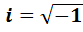• Example: We can write
•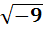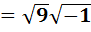= 3i

Composite

Number

• A composite number can be divided evenly by numbers other than 1 or itself.
• Examples: 4, 6, 8, 9, 10, etc.
• Multiples

• If a number is divisible exactly by a second number, then the first number is said to be a multiple of the second number.
• Example: 15 is a multiple of 5.
• Factors

• If one number divides a second number exactly, then the first number is said to be a factor of the second number.
• Example: 5 is a factor of 15.
• Even Numbers

• Numbers divisible by 2 are called even numbers.
• Examples: 2, 4, 6, 8.
• Odd

Numbers

• Numbers which are NOT divisible by 2 are called odd numbers.
• Examples: 1, 3, 5, 7.
• Roman

Numerals

• Roman numerals are numeral system of ancient Rome based on the letters of the alphabet, which are combined to signify the sum of their values.

•  I 1 V 5 X 10 L 50 C 100 D 500 M 1000

#### Place Value arrow_upward

• Place value determines the value of a digit in a number, based on the location of the digit.
• Below image is showing the place value for 25612.5.
•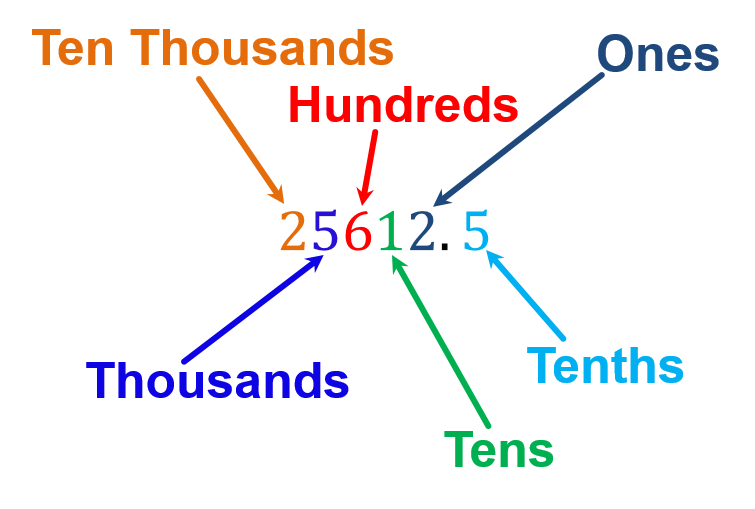• Addition is finding the total, or sum, by combining two or more numbers.
• Example: 6 + 3 = 9

• #### Subtraction arrow_upward

• The process of taking one number or amount away from another is known as subtraction.
• Example: 5 – 4 = 1

• #### Multiplication arrow_upward

• Example: 3 × 4 = 12.
• It can also be written as:
•     3 × 4 = 3 + 3 + 3 + 3 = 12

• Here 3 is the multiplicand, 4 is the multiplier and 12 is the product.

• #### Division arrow_upward

• Division is a way to find out how many times a number is contained in other number.
• Example: Division of 19 by 4.
•• Here, 4 is Divisor, 19 is Dividend, 4 is quotient, and 3 is remainder.

• #### Sign Rules arrow_upward

###### Rules for Adding Integers with the same Signs:

• Give the answer the same sign.
• Example: Add (-5) and (-10)
• Both the numbers are negative. First add the numbers.
•     5 + 10 = 15.

• As the numbers are negative so the answer would be – 15.

• ###### Rules for Adding Integers with different Signs:

• Ignore the signs and find the difference.
• Give the answer the sign of the larger number.
• Example: Add (-5) and (10)
• Ignore the signs and find the difference.
•     10 – 5 = 5

• Here the larger number 10 is positive, so the answer is 5.

• ###### Rules for Subtracting Integers:

• Change the subtraction sign to addition.
• Change the sign of the second number to the opposite sign.
• Example: Subtract: -8 – (-5).
• Change the subtraction sign to addition and change -5 to 5.
•     -8 + 5

•     = -3

• ###### Rules of Signs for Multiplication:

• When two numbers with like signs are multiplied, the result is always positive.
• When two numbers with different signs are multiplied, the result is always negative.
• Examples:
• 6 × 8 = 48
• (-6) × 8 = -48

###### Rules of Signs for Division:

• When two numbers with like signs are divided, the result is always positive.
• When two numbers with different signs are divided, the result is always negative.
• Examples:
• 36 ÷ 6 = 6
• (-36) ÷ 6 = -6

#### Order of Operations arrow_upward

• To ensure that anyone evaluating a mathematical expression calculates the same value, one has to follow the established order of operations:

•  1 Find whether a bar sign is present in the expression, evaluate it first. 2 First perform any calculations inside parentheses ( ), then brackets [ ] and braces { }. 3 Next perform any exponent operations. 4 Next perform all multiplication and division, working from left to right. If the expression contains both operations (multiplication and division), do whichever occurs first, going from left to right. 5 Lastly, perform all addition and subtraction, working from left to right.

#### Trick for remembering the Order of Operations arrow_upward

 Please First perform any calculations inside parentheses ( ), then brackets [ ] and braces { }. Excuse Next perform any exponent operations. Me Next perform all multiplication working from left to right. Dear Next perform all division, working from left to right. Aunt Next perform all addition working from left to right. Susan Lastly, perform all subtraction, working from left to right.

#### Thank You from Kimavi arrow_upward

• Please email us at Admin@Kimavi.com and help us improve this tutorial.